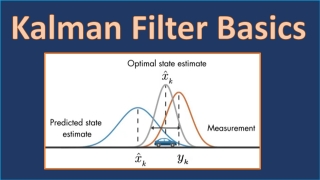# Kalman Filter BasicsExplanation 6 resources
Last Edited: 10/30/2022

This journey consists of several educational videos to introduce the basics of Kalman filtering and a virtual lab to let you practice the design and implementation of linear and extended Kalman filters.

If you want to know why engineers use Kalman filters and why this is such a popular technique for state estimation, watch this video first before moving on to the next ones below. If you instead want to dive right into the workings of Kalman filters, you can skip this video.

## Understanding Kalman Filters, Part 1: Why Use Kalman Filters?

7 min
Beginner
Video
Theory

Discover common uses of Kalman filters by walking through some examples. A Kalman filter is an optimal estimation algorithm used to estimate states of a system from indirect and uncertain...

See More

A Kalman filter is an optimal state observer. Before we get to the optimality of Kalman filters, first gain an understanding of how state observers work and discover the math behind them.

Initially, it might be difficult to grasp Kalman filter concepts if you’re starting directly with the state estimation algorithm. Instead, walk through this example which explains visually the working principle of Kalman filters.

Once you have a basic understanding of Kalman filters, you can watch this video to learn how Kalman filter algorithm computes the equations associated with the prediction and update steps.

## Understanding Kalman Filters, Part 4: An Optimal State Estimator Algorithm

9 min
Beginner
Video
Theory

Discover the set of equations you need to implement a Kalman filter algorithm. You’ll learn how to perform the prediction and update steps of the Kalman filter algorithm, and you’ll see how...

See More

A Kalman filter is defined for linear systems only so how do we deal with state estimation of real life systems as they mostly exhibit nonlinear behavior? There are different variations of Kalman filters such as Extended, Unscented Kalman filters and Particle filters that you can use for state estimation of nonlinear systems.

Try it yourself! Download the Kalman filter virtual lab and practice design of a linear and extended Kalman filters using a simple pendulum example. You’ll be able to test your design with simulation and pendulum animations.